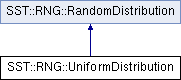SST  11.1.0 StructuralSimulationToolkit
SST::RNG::UniformDistribution Class Reference

Creates a Uniform distribution for use within SST. More...

`#include "sst/core/rng/uniform.h"`

Inheritance diagram for SST::RNG::UniformDistribution:## Public Member Functions

UniformDistribution (const uint32_t probsCount)
Creates an uniform distribution with a specific number of bins. More...

UniformDistribution (const uint32_t probsCount, SST::RNG::Random *baseDist)
Creates a Uniform distribution with a specific number of bins and user supplied random number generator. More...

~UniformDistribution ()
Destroys the distribution and will delete locally allocated RNGs.

double getNextDouble ()
Gets the next (random) double value in the distribution. More...Public Member Functions inherited from SST::RNG::RandomDistribution
virtual ~RandomDistribution ()
Destroys the distribution.

RandomDistribution ()
Creates the base (abstract) class of a distribution.

## Protected Attributes

SST::RNG::RandombaseDistrib
Sets the base random number generator for the distribution.

const bool deleteDistrib
Controls whether the base distribution should be deleted when this class is destructed.

const uint32_t probCount
Count of discrete probabilities.

double probPerBin
Range 0..1 split into discrete bins.

## Detailed Description

Creates a Uniform distribution for use within SST.

This distribution is the same across platforms and compilers.

## Constructor & Destructor Documentation

 SST::RNG::UniformDistribution::UniformDistribution ( const uint32_t probsCount )
inline

Creates an uniform distribution with a specific number of bins.

Parameters
 probsCount Number of probability bins in this distribution
 SST::RNG::UniformDistribution::UniformDistribution ( const uint32_t probsCount, SST::RNG::Random * baseDist )
inline

Creates a Uniform distribution with a specific number of bins and user supplied random number generator.

Parameters
 probsCount Number of probability bins in the distribution baseDist The base random number generator to take the distribution from.

## Member Function Documentation

 double SST::RNG::UniformDistribution::getNextDouble ( )
inlinevirtual

Gets the next (random) double value in the distribution.

Returns
The next random double from the distribution, this is the double converted of the index where the probability is located

Implements SST::RNG::RandomDistribution.

The documentation for this class was generated from the following file: